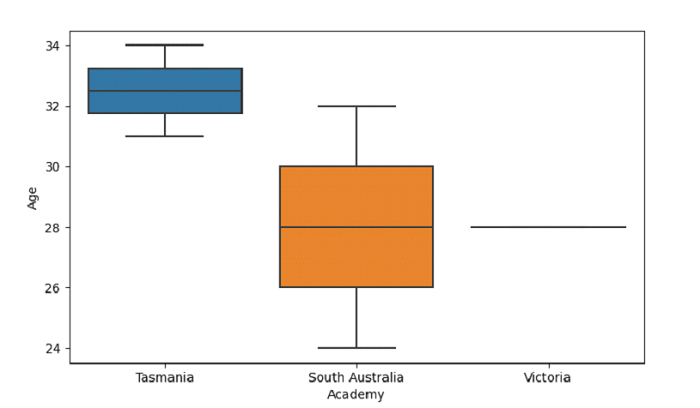# Python Pandas - Draw a boxplot and control box order by passing an explicit order with Seaborn

Box Plot in Seaborn is used to draw a box plot to show distributions with respect to categories. The seaborn.boxplot() is used for this. To control the order use the order parameter.

Let’s say the following is our dataset in the form of a CSV file − Cricketers.csv

At first, import the required libraries −

import seaborn as sb
import pandas as pd
import matplotlib.pyplot as plt

Load data from a CSV file into a Pandas DataFrame −

dataFrame = pd.read_csv("C:\Users\amit_\Desktop\Cricketers.csv")


Plotting box plot with Academy and Age. Control box order by passing an explicit order i.e. ordering on the basis of "Academy". Ordering using the order parameter −

sb.boxplot( x = 'Academy',y = 'Age', data = dataFrame, order=["Tasmania", "South Australia", "Victoria"] )

## Example

Following is the code −

import seaborn as sb
import pandas as pd
import matplotlib.pyplot as plt

# Load data from a CSV file into a Pandas DataFrame

# plotting box plot with Academy and Age
# Control box order by passing an explicit order i.e. ordering on the basis of "Academy"
# ordering using the order parameter
sb.boxplot( x = 'Academy',y = 'Age', data = dataFrame, order=["Tasmania", "South Australia", "Victoria"] )

# display
plt.show()

## Output

This will produce the following output −Updated on: 04-Oct-2021

504 Views